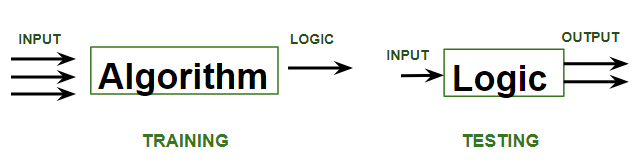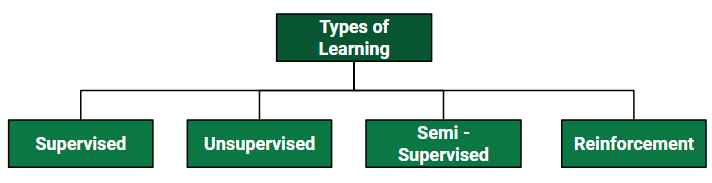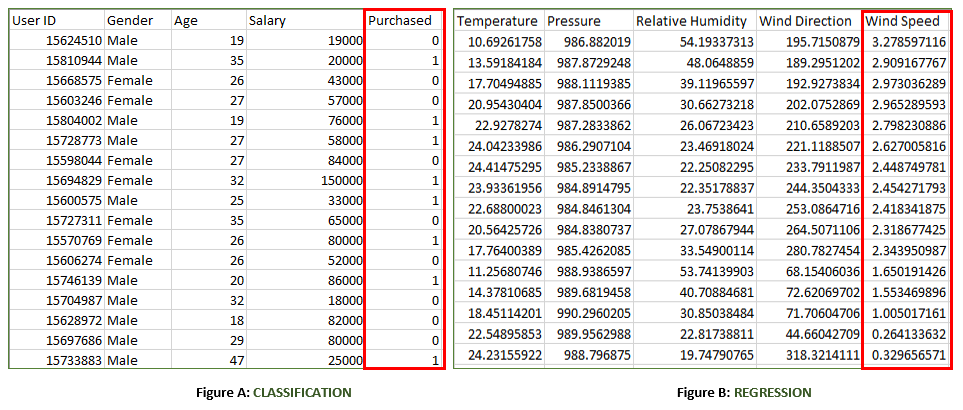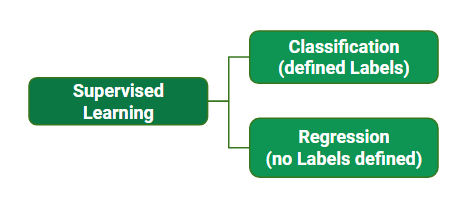# ML | Types of Learning – Supervised Learning

• Difficulty Level : Easy
• Last Updated : 13 Sep, 2021What is Learning for a machine?

A machine is said to be learning from past Experiences(data feed in) with respect to some class of tasks if its Performance in a given Task improves with the Experience. For example, assume that a machine has to predict whether a customer will buy a specific product let’s say “Antivirus” this year or not. The machine will do it by looking at the previous knowledge/past experiences i.e the data of products that the customer had bought every year and if he buys Antivirus every year, then there is a high probability that the customer is going to buy an antivirus this year as well. This is how machine learning works at the basic conceptual level.Supervised Learning :
Supervised learning is when the model is getting trained on a labelled dataset. A labelled dataset is one that has both input and output parameters. In this type of learning both training and validation, datasets are labelled as shown in the figures below.Both the above figures have labelled data set –

• Figure A: It is a dataset of a shopping store that is useful in predicting whether a customer will purchase a particular product under consideration or not based on his/ her gender, age, and salary.
Input: Gender, Age, Salary
Output: Purchased i.e. 0 or 1; 1 means yes the customer will purchase and 0 means that the customer won’t purchase it.
• Figure B: It is a Meteorological dataset that serves the purpose of predicting wind speed based on different parameters.
Input: Dew Point, Temperature, Pressure, Relative Humidity, Wind Direction
Output: Wind Speed

Training the system:
While training the model, data is usually split in the ratio of 80:20 i.e. 80% as training data and rest as testing data. In training data, we feed input as well as output for 80% of data. The model learns from training data only. We use different machine learning algorithms(which we will discuss in detail in the next articles) to build our model. By learning, it means that the model will build some logic of its own.
Once the model is ready then it is good to be tested. At the time of testing, the input is fed from the remaining 20% data which the model has never seen before, the model will predict some value and we will compare it with actual output and calculate the accuracy.Types of Supervised Learning:

1. Classification: It is a Supervised Learning task where output is having defined labels(discrete value). For example in above Figure A, Output – Purchased has defined labels i.e. 0 or 1; 1 means the customer will purchase and 0 means that customer won’t purchase. The goal here is to predict discrete values belonging to a particular class and evaluate them on the basis of accuracy.
It can be either binary or multi-class classification. In binary classification, the model predicts either 0 or 1; yes or no but in the case of multi-class classification, the model predicts more than one class.
Example: Gmail classifies mails in more than one class like social, promotions, updates, forums.
2. Regression: It is a Supervised Learning task where output is having continuous value.
Example in above Figure B, Output – Wind Speed is not having any discrete value but is continuous in the particular range. The goal here is to predict a value as much closer to the actual output value as our model can and then evaluation is done by calculating the error value. The smaller the error the greater the accuracy of our regression model.

Example of Supervised Learning Algorithms:

• Linear Regression
• Nearest Neighbor
• Gaussian Naive Bayes
• Decision Trees
• Support Vector Machine (SVM)
• Random Forest
My Personal Notes arrow_drop_up